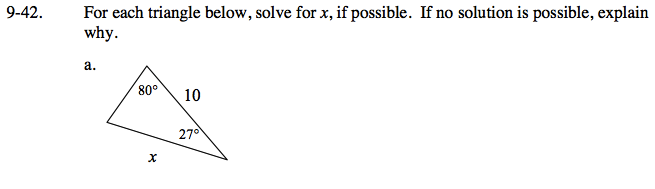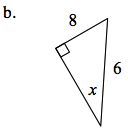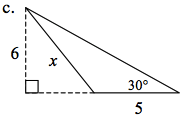### Home > GC > Chapter 9 > Lesson 9.1.4 > Problem9-42

9-42.Which law can you use to solve for x?

180° = 80° + 27° + y, where y is the unknown angle.

y = 73°

Use the Law of Sines to find the length of x.

$\frac{\text{sin}80}{x}=\frac{\text{sin}73}{10}$

x ≈ 10.3

Which side is the longest side of this triangle?
Is that possible?

Find the length of the whole base (including the dotted line). How can you use this to find x?

x ≈ 8.07